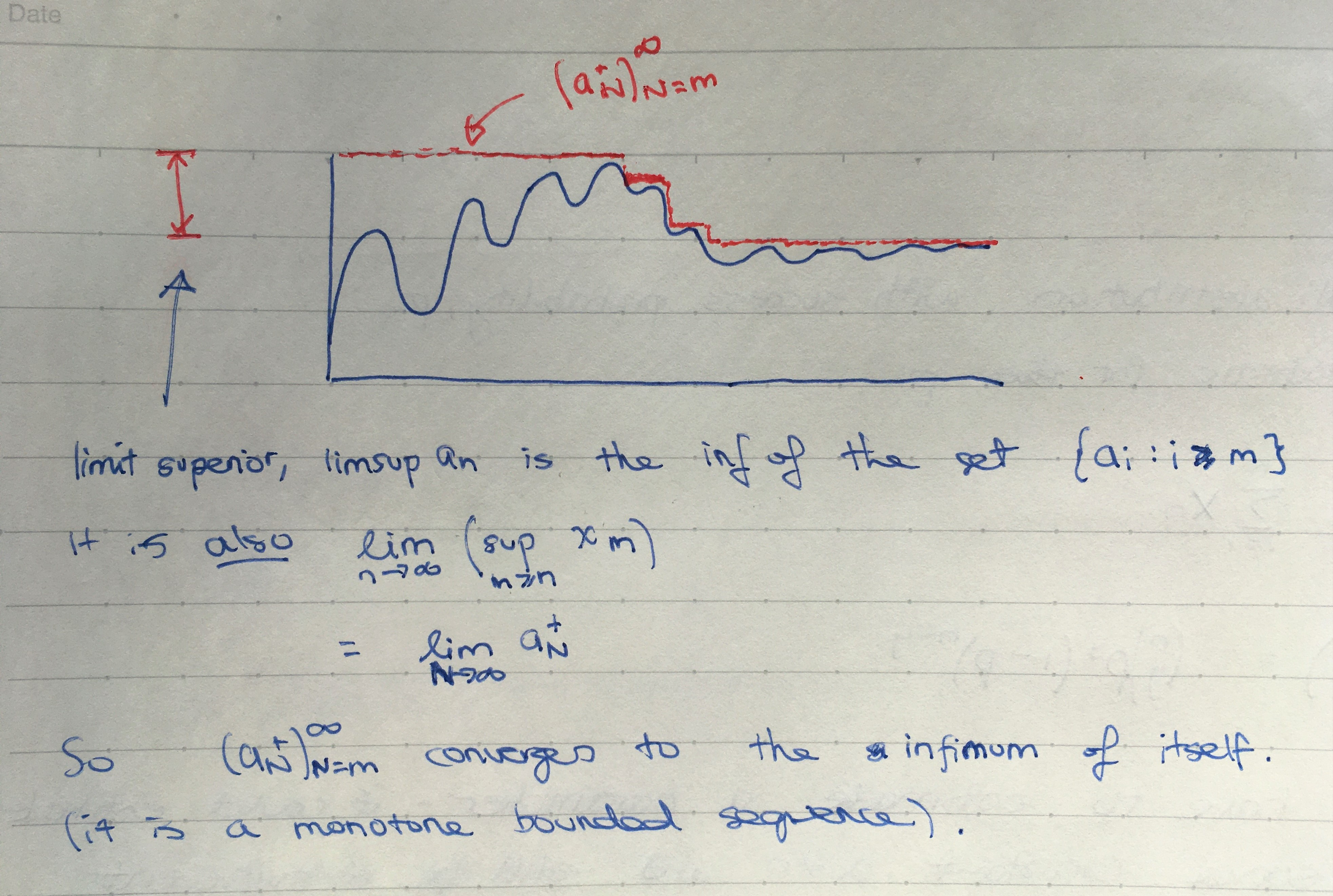Show Question
Math and science::Analysis::Tao::06. Limits of sequences

# Limit superior and limit inferior

Suppose that $$(a_n)_{n=m}^{\infty}$$ is a sequence. We define a new sequence $$(a_N^+)_{N=m}^{\infty}$$ by the formula:

$a_N^+ := \sup(a_n)_{n=N}^{\infty}$

In other words, $$a_N^+$$ is the supremum of all the elements in the sequence from $$a_N$$ onwards.

We then define the limit superior of the sequence $$(a_n)_{n=m}^{\infty}$$, denoted by $$\limsup_{n\rightarrow \infty}a_n$$, by the formula:

$\limsup_{n\rightarrow \infty}a_n := \inf(a_N^+)_{N=m}^{\infty}$

The mirror proceedure defines the limit inferior. First define:

$a_N^- := \inf(a_n)_{n=N}^{\infty}$

The the limit inferior is:

$\liminf_{n\rightarrow \infty}a_n := \sup(a_N^-)_{N=m}^{\infty}$

The above formulations present limit superior as the smallest supremum of all sequences with different starting points. Ditto limit inferior. Limit superior and inferior can be defined instead as limits (which makes their name make more sense):

$\limsup_{n\rightarrow \infty} a_n := \lim_{n\rightarrow \infty} ( \sup_{m\gt n} x_m )$

Limit superior/inferior, using Tao's piston analogy, are the points where pistons pressing down (superior) and up(inferior) on a sequence will come to rest as the pistons are moved towards infinity along the tail of the sequence.

### Example

A sequence with a cosine shape is an example that demonstrates an clear difference between limit points and limit inferior/superior. A constant magnitude cosine will have every value from [-1,1] as a limit point. 1 will be the limit superior, and -1 will be the limit inferior.

Another example: